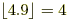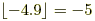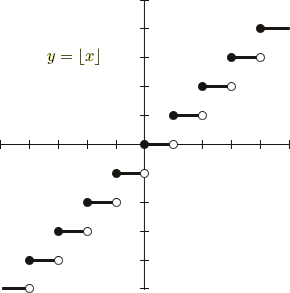index: click on a letter A B C D E F G H I J K L M N O P Q R S T U V W X Y Z A to Z index index: subject areas numbers & symbols sets, logic, proofs geometry algebra trigonometry advanced algebra & pre-calculus calculus advanced topics probability & statistics real world applications multimedia entrieswww.mathwords.com about mathwords website feedback

 Floor Function Greatest Integer Function A step function of x which is the greatest integer less than or equal to x. The floor function is written a number of different ways: with special bracketsor, or by using either boldface brackets [x] or plain brackets [x]. Examples:and.See also Ceiling function (also known as least integer function)